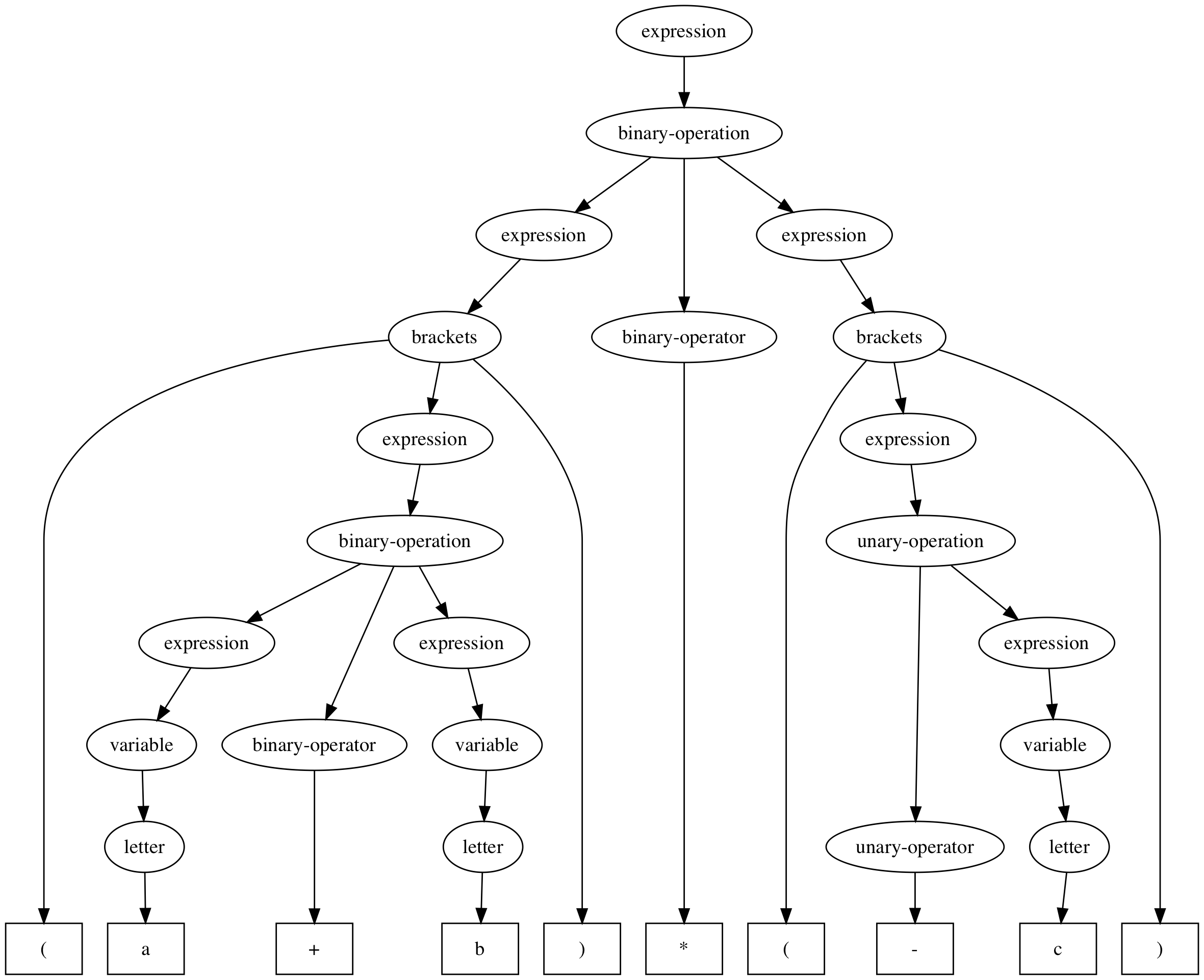# Covfefe

Covfefe is a parser framework for languages generated by any (deterministic or nondeterministic) context free grammar. It implements the Earley and CYK algorithm.

## Usage

### Swift Package Dependency in Xcode

1. Go to “File” > “Swift Packages” > “Add Package Dependency…”
2. Enter “https://github.com/palle-k/Covfefe.git” as the repository URL.
3. Select “Version”, “Up to next major”, “0.6.1” < “1.0.0”

### Swift Package Manager

This framework can be imported as a Swift Package by adding it as a dependency to the `Package.swift` file:

``````.package(url: "https://github.com/palle-k/Covfefe.git", from: "0.6.1")
``````

### CocoaPods

Alternatively, it can be added as a dependency via CocoaPods (iOS, tvOS, watchOS and macOS).

``````target 'Your-App-Name' do
use_frameworks!
pod 'Covfefe', '~> 0.6.1'
end
``````

## Example

Grammars can be specified in a superset of EBNF or a superset of BNF, which adopts some features of EBNF (documented here). Alternatively, ABNF is supported.

``````let grammarString = """
expression       = binary-operation | brackets | unary-operation | number | variable;
brackets         = '(', expression, ')';
binary-operation = expression, binary-operator, expression;
binary-operator  = '+' | '-' | '*' | '/';
unary-operation  = unary-operator, expression;
unary-operator   = '+' | '-';
number           = {digit};
digit            = '0' ... '9';
variable         = {letter};
letter           = 'A' ... 'Z' | 'a' ... 'z';
"""
let grammar = try Grammar(ebnf: grammarString, start: "expression")
``````

This grammar describes simple mathematical expressions consisting of unary and binary operations and parentheses. A syntax tree can be generated, which describes how a given word was derived from the grammar above:

``````let parser = EarleyParser(grammar: grammar)

let syntaxTree = try parser.syntaxTree(for: "(a+b)*(-c)")
``````